# Switching Buck Regulator: Design Basics and Efficiency

Published  August 6, 2018   0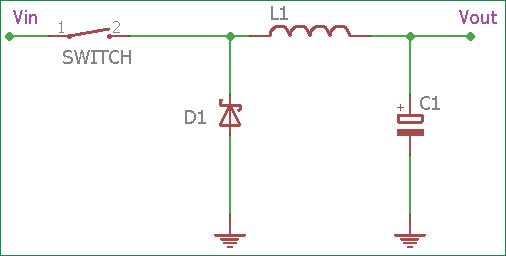In electronics, a regulator is a device or mechanism which can regulate power output constantly. There are different kinds of regulators available in the power supply domain. But mainly, in the case of DC to DC conversion, there are two types of regulators available: Linear or Switching.

A linear regulator regulates the output using a resistive voltage drop and due to this Linear regulators provide lower efficiency and lose power in the form of heat.

On the other side Switching regulator use inductor, Diode and a power switch to transfer energy from its source to the output.

There are three types of switching regulators available.

1.Step-up  converter (Boost Regulator)

2.Step-Down converter (Buck regulator)

3.Inverter (Flyback)

In this tutorial, we will describe the Switching Buck Regulator circuit. We already described the Buck Regulator Design in the previous tutorial. Here we will discuss different aspects of Buck converter and how to improve its efficiency.

### Difference between Buck and Boost Regulator

The difference between the buck and boost regulator is, in the buck regulator the placement of inductor, diode and the switching circuit is different than the boost regulator. Also, in case of boost regulator the output voltage is higher than the input voltage, but in buck regulator, the output voltage is lower than the input voltage.

A buck topology or buck converter is one of the most used basic topology used in SMPS. It’s a popular choice where we need to convert higher voltage to a lower output voltage.

Same as the boost regulator, a buck converter or buck regulator consist of an inductor, but the connection of the inductor is in output stage rather than the input stage used in boost regulators.

So, in many cases, we need to convert lower voltage to the higher voltage depending on the requirements. Buck regulator converts the voltage from higher potential to lower potential.

### Design Basics of Buck Converter CircuitIn the above image, a simple Buck regulator circuit is shown where an Inductor, diode, Capacitor and a switch are used. The input is directly connected across the switch. The Inductor and capacitor are connected across the output, thus the load gets smooth output current waveform. The diode is used for blocking the negative current flow.

In case of switching boost regulators, there are two Phases, One is Inductor Charge phase or the Switch-on phase (Switch is closed actually) and the other one is Discharge phase or the switch-off phase (Switch is open).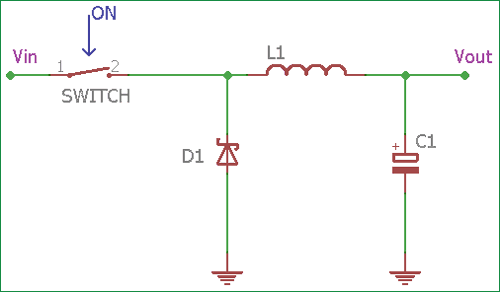If we assume that the switch has been in open position for a long time, the current in the circuit is 0 and there is no voltage present.

In this situation, if the switch gets close then the current will increase and the inductor will create a voltage across it. This voltage drop minimizes the source voltage at the output, after a few moments the rate of current change decrease and the voltage across the inductor also decrease which eventually increase the voltage across the load. Inductor store energy using it’s magnetic field.

So, when the switch is on, across the inductor the voltage is VL = Vin - Vout

The current in the inductor rise at a rate of (Vin – Vout) / L

The current through the inductor rises at linearly with time. The linear current rising rate is proportional to the input voltage less output voltage divided by the inductance

`di / dt = (Vin - Vout) / L`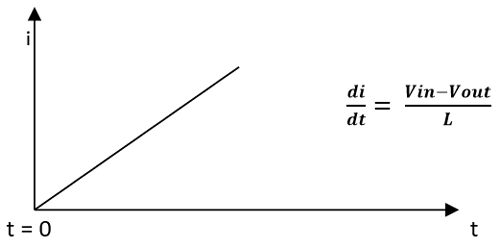The upper graph showing the Charging phase of the inductor. The x-axis denotes t (time) and the Y-axis denotes i (current through the inductor). The Current is increasing linearly with time when the switch is closed or ON.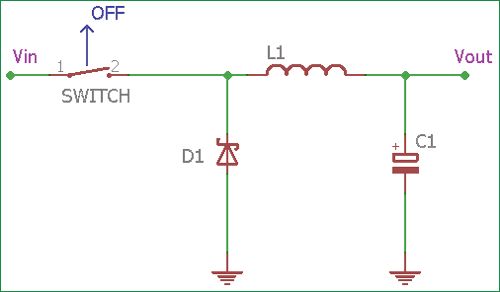Now if the switch will open during this time while the current is still changing, there will always a voltage drop occurring across the inductor. The voltage across the load will be lower than the input voltage. During the off state, while the switch is open, input voltage source gets disconnected, and the inductor will transfer the stored energy to the load. The inductor will become the current source for the load.

The Diode D1 will provide a return path of the current flowing through the inductor during the switch off-state.

The inductor current decrease with a slope equal to –Vout / L

### Buck Converter Operating Modes

The Buck converter can be operated in two different modes. Continuous mode or discontinuous mode.

Continuous mode

During Continuous mode, the inductor never discharged fully, the charging cycle starts when the inductor is partially discharged.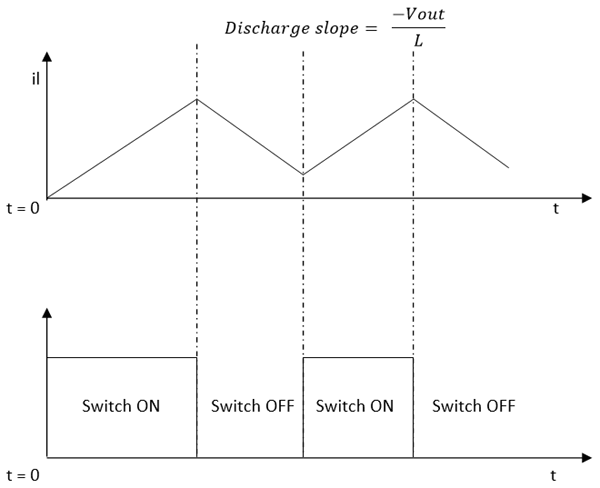In the above image, we can see, when the switch gets on when inductor current (iI) increase linearly, then when the switch gets off the inductor start to decrease, but the switch again gets on while the inductor is partly discharged. This is the Continuous mode of operation.

Energy stored in the inductor is E = (LI L2) / 2

Discontinuous Mode

Discontinuous mode is slightly different than the continuous mode. In the Discontinuous mode, the Inductor discharged fully before starting a new charge cycle. The Inductor will discharge fully to the zero before the switch became on.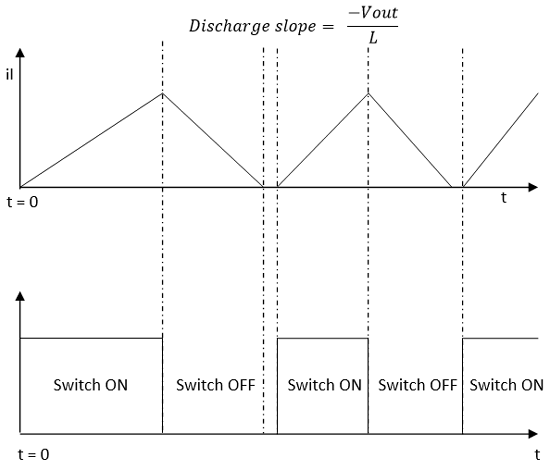During discontinuous mode, as we can see in the above image when the switch gets on the, the inductor current (il) increase linearly, then when the switch gets off, the inductor starts to decrease, but the switch only gets on after the inductor is fully discharged and the inductor current became completely zero. This is the Discontinuous mode of operation. In this operation, the current flow through the inductor is not continuous.

### PWM and Duty Cycle for Buck Converter Circuit

As we discussed in previous buck converter tutorial, varying the duty cycle we can control buck regulator circuit. For this, a basic control system is required. An Error amplifier and switch control circuit is additionally required which will work in continuous or discontinuous mode.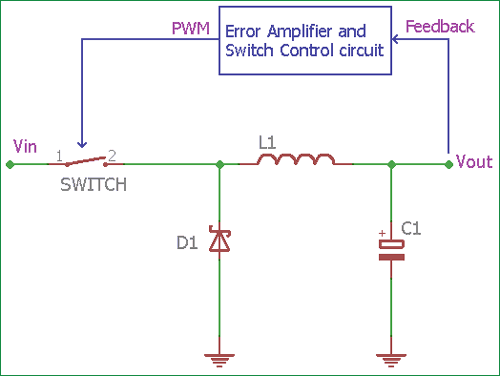So, for a complete buck regulator circuit, we need an additional circuitry which will vary the duty cycle and thus the amount of time the inductor receives energy from the source.

In the above image, an Error amplifier can be seen which sense the output voltage across the load using a feedback path and control the switch. Most common control technic includes PWM or Pulse Width Modulation technic which is used to control the duty cycle of the circuitry.

The control circuit controls the amount of time the switch remain open or, controlling how much time the inductor charge or discharge.

This circuit controls the switch depending on the mode of operation. It will take a sample of the output voltage and to subtract it from a reference voltage and create a small error signal, then this error signal will be compared to an oscillator ramp signal and from the comparator output a PWM signal will operate or control the switch circuit.

When the output voltage changes, the error voltage also affected by it. Due to error voltage change, the comparator controls the PWM output. The PWM also changed to a position when the output voltage creates zero error voltage and by doing this, the closed control loop system executes the work.

Fortunately, Most modern Switching buck regulators have this thing inbuilt inside the IC package. Thus simple circuitry design is achieved using the modern switching regulators.

The reference feedback voltage is done using a resistor divider network. This is the additional circuitry, which is needed along with inductor, diodes, and capacitors.

### Improve efficiency of Buck Converter Circuit

Now, if we investigate about the efficiency, how much power we provide inside the circuitry and how much we get at the output. (Pout / Pin) * 100%

As energy cannot be created nor destroyed, it can only be converted, most electrical energies loose unused powers converted to heat. Also, there is no ideal situation in the practical field, efficiency is a larger factor for selecting Voltage regulators.

One of the main power-loss factors for a switching regulator is the diode. The forward voltage drop multiply by current (Vf x i) is the unused wattage which is converted to heat and reduces the efficiency of the switching regulator circuit. Also, it is the additional cost to the circuitry for thermal/heat management technics using a heatsink, or Fans to cool down the circuitry from dissipated heat. Not only the forward voltage drop, Reverse recovery for silicon diodes also produce unnecessary power loss and reduction of the overall efficiency.

One of the best way to avoid a standard recovery diode is to use Schottky diodes in place of diodes that have a low forward voltage drop and better reverse recovery. When maximum efficiency is needed, the diode can be replaced using MOSFETs. In modern technology, there are plenty of choices available in Switching buck regulator section, which provide more than 90% efficiency easily.

Despite having Higher efficiency, Stationary design technic, smaller component, switching regulators are noisy than a linear regulator.  Still, they are widely popular.

### Example Design for Buck Converter

We previously created a buck regulator circuit using MC34063 where the 5V output is generated from the 12V input voltage. MC34063 is the switching regulator which was used in buck regulator configuration. We used an Inductor, a Schottky diode, and capacitors.In the above image, Cout is the output capacitor and we also used an inductor and Schottky diode which are the basic components for a switching regulator. There is also a Feedback network used. R1 and R2 resistors create a voltage divider circuit which is needed for the comparator's PWM and error amplification stage. The reference voltage of the comparator is 1.25V.

If we see the project in detail, we can see that 75-78% efficiency is achieved by this MC34063 switching buck regulator circuit. Further efficiency can be improved using proper PCB technic and obtaining thermal management procedures.

Example Use of Buck regulator-

1. Dc power source in the low voltage application
2. Portable equipment
3. Audio equipment
4. Embedded hardware systems.
5. Solar systems etc.